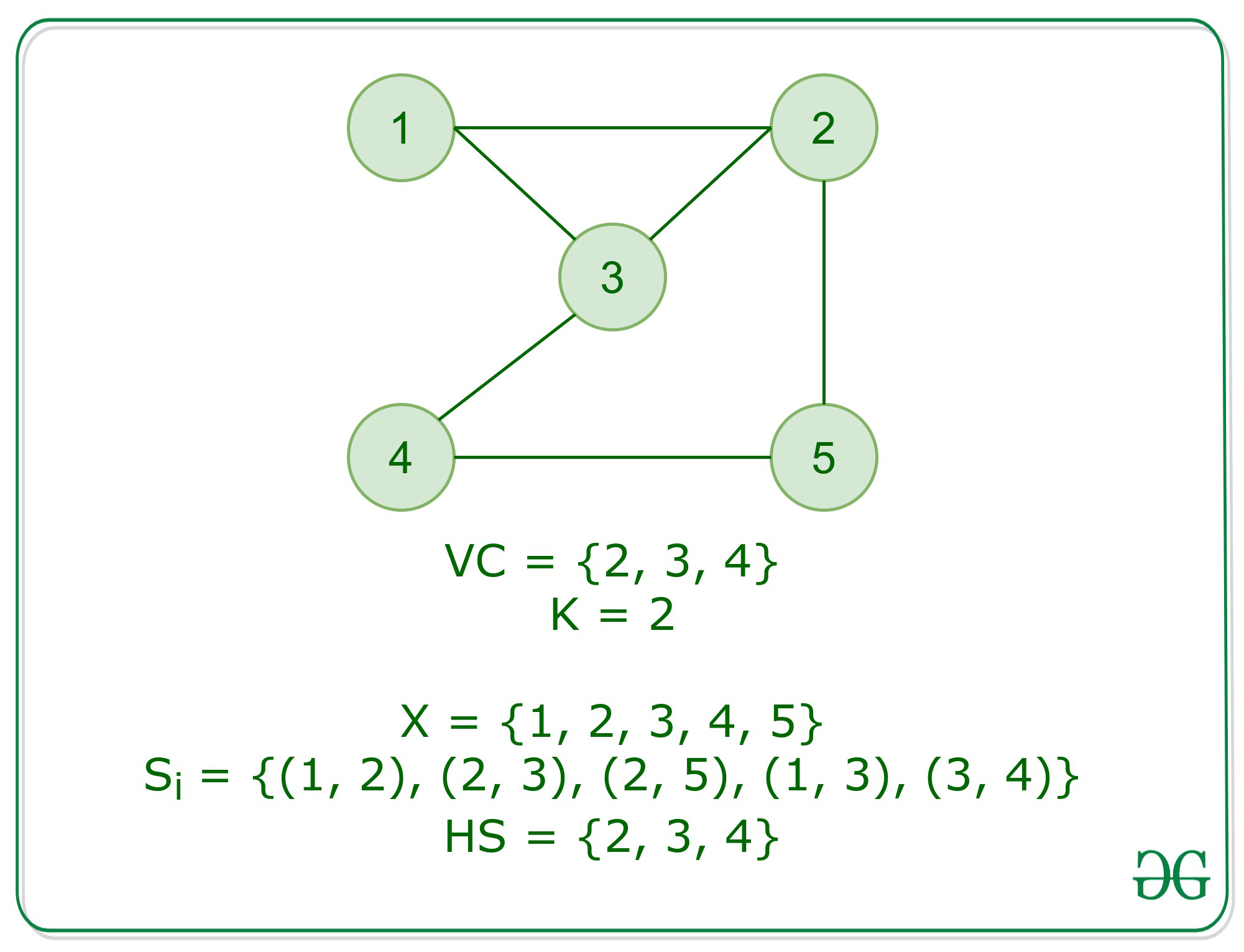Related Articles

# Hitting Set problem is NP Complete

• Difficulty Level : Easy
• Last Updated : 06 Oct, 2020

Prerequisite: NP Complete

Problem: Given a ground set X of elements and also a grouping collection C of subsets available in X and an integer k, the task is to find the smallest subset of X, such that the smallest subset, H hits every set comprised in C. This implies that the intersection of H and S is null for every set S belonging to C, with size ≤ k.

Proof: An instance of the problem is an input specified to the problem. An instance of the Hitting Set is a collection C of subset, S in X, and k. Since an NP-complete problem, by definition, is a problem which is both NP and NP-Hard, the proof or statement that a problem is NP-Complete consists of two parts:

1. The problem itself is NP-Complete.
2. All other problems in NP class can be polynomial-time reducible to that. (B is poly-time reducible to C).

If the only second condition is satisfied, the problem is called NP-Hard.
But it is not possible to reduce every NP problem into another NP problem to show its NP-Completeness all the time. That is why to show a problem is NP-complete, prove that the problem is in NP and any NP-Complete problem is reducible to that then we are done. Thus, it can be verified that the hitting set problem is NP-Complete using the following propositions:

1. Hitting Set is in NP: It any problem is in NP, then given a ‘certificate’, which is a solution to the problem and an instance of the problem (a ground set X, a collection, C of subsets, S), we will be able to verify (check whether the solution is correct or not) the certificate in polynomial time. This can be done by:
Provided a Hitting Set, HS of size k, verify it covers at least one element in every set Si of X.
This takes polynomial time, hence in NP
2. Hitting Set is NP-Hard: In order to prove Hitting Set is NP-Hard, we will perform a reduction from which vertex cover problem can be reduced to the Hitting Set problem.

In the Vertex Cover problem, we have a graph G = (V, E)
Now, Let X, that is the ground set = vertices of G. That is X = V(G) and the collection C of subset Si in X is Si = {u, v} is an edge in graph G.Now, the following properties hold:

• If VC is the vertex cover of graph G of size k, this implies that for every edge {u, v} either u or v belongs to VC. Therefore, VC forms the Hitting Set because all the subsets will form an intersection with the vertices in VC.
• If HS is hitting a set of X of size k. Now since HS intersects every subset of X, at least one of the endpoints of every edge {u, v} must belong to the solution. Therefore, it spans at least one vertex for every edge, Hence forming VC.

Conclusion:

The Hitting Set Problem is NP and NP-Hard. Therefore, the Hitting Set Problem is NP-Complete.

Attention reader! Don’t stop learning now. Get hold of all the important DSA concepts with the DSA Self Paced Course at a student-friendly price and become industry ready.  To complete your preparation from learning a language to DS Algo and many more,  please refer Complete Interview Preparation Course.

In case you wish to attend live classes with experts, please refer DSA Live Classes for Working Professionals and Competitive Programming Live for Students.

My Personal Notes arrow_drop_up